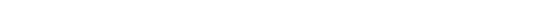NEWS NLREG has been selected as the "Editor"s Pick" by SoftSeek.NLREG is in use at hundreds of universities, laboratories, and government agencies around the world (over 20 countries). For a list of a few organizations using NLREG click here. If you have categorical variables, you may want to use a Decision Tree to model your data. Check out the DTREG Decision Tree Builder. You also should check out the News Rover program that automatically scans Usenet newsgroups, downloads messages of interest to you, decodes binary file attachments, reconstructs files split across multiple messages, and eliminates spam and duplicate files.## NLREG Function and Surface Plots

In addition to fitting a function to a set of data values, NLREG can generate various plots showing the fit or providing information about the quality of the fit.

### Function Plot

A function plot displays the actual data values as X-marks and shows how the fitted function aligns with the data points. Optionally, the plot can show vertical lines between the data points and the function curve representing the residual (error) values for each point. It is also possible to specify a domain over which the function will be plotted allowing it to be extrapolated in either direction beyond the domain of the data values.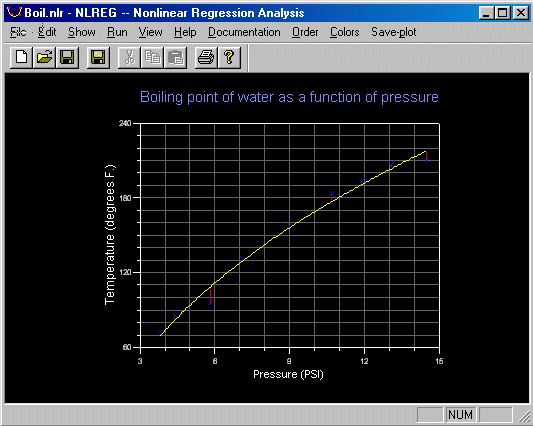### 3D Surface Plot

The Advanced version of NLREG supports 3D surface plots. Surface plots depict the value of the dependent variable on the Z axis and two independent variables on the X and Y axes. Circles are used to show the actual data points. Optionally, contour lines can be shown on the base surface below the figure.### Surface Contour Plot

The Advanced version of NLREG supports surface contour plots. Contour plots depict the value of the dependent variable as color-coded regions and the values of two independent variables on the X and Y axes.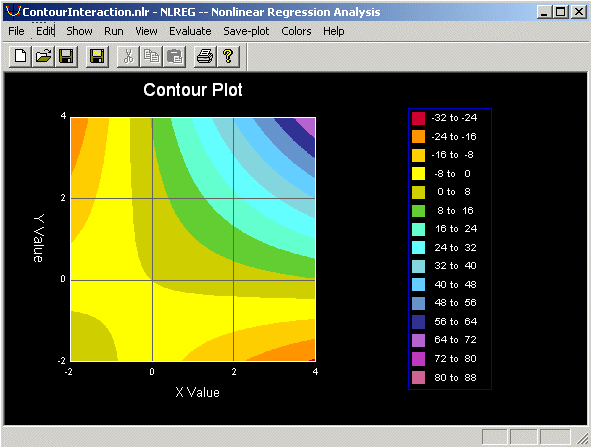### Surface Residual Plot

The Advanced version of NLREG can generate 3D surface residual plots showing the computed and actual value of each data point for functions with two independent variables. The computed and actual points are shown as circles with a line connecting them. Optionally, a contour plot of the function values can be shown on the surface of the plot.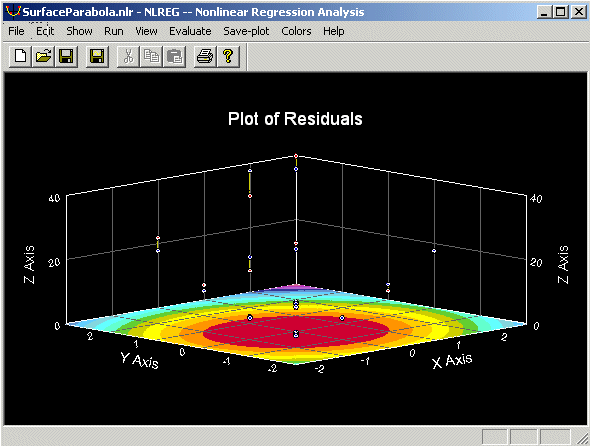### Function Residual Plot

This type of plot shows the residual (error) values. That is, each Y value corresponds to the difference between the actual value of a data point and the computed value of the fitted function at the corresponding X value. It is useful for identifying data points that deviate significantly from the fitted function.### Normal Probability Plot

The actual value of each residual is plotted on the vertical (Y) axis and the expected value of the residual, assuming the residuals are normally distributed, is plotted on the horizontal (X) axis. If the residuals are normally distributed, the resulting plot will be a straight line passing through the origin with a slope of 1. A normal probability plot is useful for determining whether the residuals are normally distributed. If they are not normally distributed then the form of the function being fitted may be inappropriate for the data.### Controlling Plot Colors

NLREG allows you to specify the colors of various items in a plot. Three sets of color values are stored: (1) colors for plots displayed on your screen, (2) colors for plots that you print, and (3) colors for plots saved to disk files.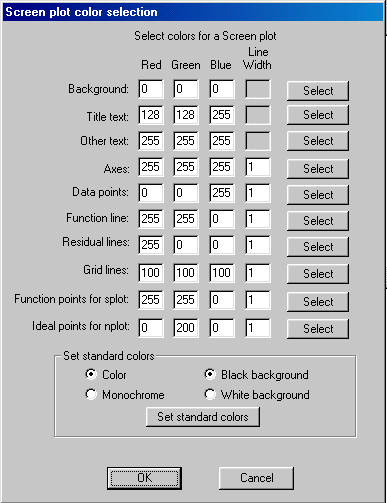### Saving Plots to Disk Files

NLREG makes it easy to save plot images to disk files so that you can import them into documents. Once you get a plot that you want to save, just click the "Save-plot" button and specify the name of the file, the size of the plot and whether you want it saved as a JPG image or a BMP image.